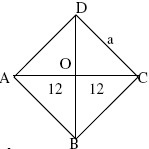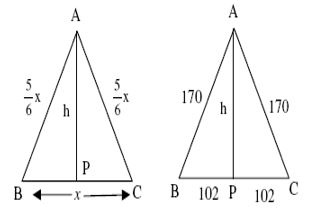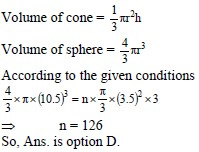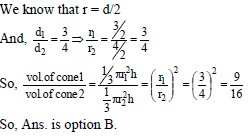# Sample Aptitude Questions of SBI

1. The area of a rhombus is 216 cm2 and the length of its one diagonal is 24 cm. The perimeter ( in cm) of the rhombus
1. 52
2. 60
3. 120
4. 100

Area of rhombus = 1/2 × d1 × d2 where d1 and d2 are lengths of diagonal 1 and 2
So, 216 = 1/2 × 24 × d2
=> d2 = 18cmIn ▲DOC, a2 + ( 18/2 )2 = 144 + 81 = 225
Therefore, a = √225 = 15cm
So, perimeter = 4a = 4 × 15 = 60cm
So, Ans. is option B
2. The perimeter of an isosceles triangle is 544 cm and each of the equal sides is 5/6 times the base. What is the area (in cm2) of the triangle?
1. 38172
2. 18372
3. 31872
4. 13872

Perimeter = 544x + 5x/6 + 5x/6 = 544
Therefore x = 204
Let. AB = AC = a = 5x/6
=> a = 5/6 × 204, A = 170
Now, in ▲APC, 1702 = h2 + 1022
h2 = 1702 - 1022
=> h = √18496 = 136
=> area of ▲ABC = 1/2 × 204 × 136 = 13872 cm2
1. A metallic sphere of radius 10.5 cm is melted and then recast into small cones each of radius 3.5 cm and height 3 cm. The number of cones thus formed is
1. 140
2. 132
3. 112
4. 1262. If the ratio of the diameters of two right circular cones of equal height be 3: 4, then the ratio of their volumes will be
1. 3:4
2. 9:16
3. 16:9
4. 27:643. The sum of two numbers is equal to 15 and their arithmetic mean is 25 per cent greater than their geometric mean. Find the numbers.
1. 5 & 10
2. 3 & 12
3. 1 & 14
4. 6 & 9
5. None of these

AM of 2 numbers is a+b/2.GM of 2 numbers is √a+b.When sum of 2
numbers is 15, their AM is 7.5. AM = 1.25 (GM)
=> GM =7.5/1.25 = 6. Hence 36 = ab. So product of 2 numbers is 36. Try by
4. The product of the digits of a two-digit number is twice as large as the sum of its digits. If we subtract 27 from the required number, we get a number consisting of the same digits written in the reverse order. Find the number.
1. 36
2. 27
3. 63
4. 88
5. None of these

Go by options. 3rd option is the answer because 63 => product of digits = 6*3 = 18.
Sum of digits = 6 + 3 = 9. Hence product of digits is twice as the sum of the digits. Also 63 – 27 = 36.
So digits are reversed.
5. The average weight of 8 person's increases by 2.5 kg when a new person comes in place of one of them weighing 65 kg. What will be the weight of the new person?
1. 76
2. 76.5
3. 85
4. 80
5. 90

Increased wt. of 8 persons = 8 × 2.5 = 20 kg
wt. of new person = 65 + 20 = 85 kg
6. 50% of a number is 18 less than two-third of that number. Find the number.
1. 123
2. 115
3. 119
4. 108
5. 101

Let the no. be x
Given : 50x/100 = 2x/3 – 18
½ x – 2/3 x = - 18
=> x = 108
7. Running at the same constant rate, 6 identical machines can produce a total of 270 bottles per minute. At this rate, how many bottles could 10 such machines produce in 4 minutes?
1. 648
2. 1800
3. 2700
4. 2000
5. 3080

Let the no. of bottles be ‘B’. Using chain rule:
(6×1)/270 = (10×4)/B => B = 1800
8. At 60% of its usual speed, a train of length L meters crosses platform 240 meters long in 15 seconds. At its usual speed, the train crosses a pole in 6 seconds. What is the value of L (in meters)?
1. 140
2. 225
3. 220
4. 480
5. 240